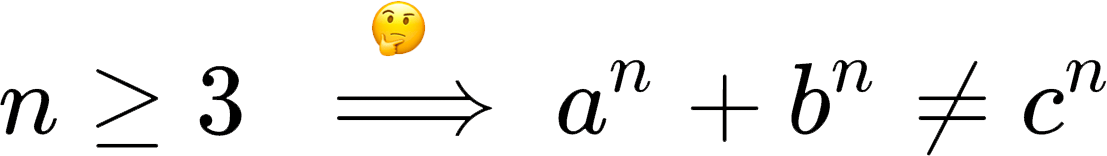# Top 10 emoji for use in mathematics

We take a look at the top 10 emojis!Maths loves symbols. Everyone loves emoji. It’s 2017 and time we brought the two together. To get you started, here are our top ten emoji for use in mathematics!

## 10.

?

Don’t leave home without one: it’s the nifty 45° set square. What better reminder is there that
$$\sin\left(\frac{\pi}{4}\right) = \frac{\sqrt{2}}{2}, \qquad \cos\left(\frac{\pi}{4}\right) = \frac{\sqrt{2}}{2}, \qquad \tan\left(\frac{\pi}{4}\right) = 1 \quad ?$$

## 9.

?

Perfect for popping over a letter to make it a vector, it’s the bow and arrow:## 8.

◼️

You can’t argue with this, it’s the QED proof square:

$\displaystyle\implies \Re[s] = \frac{1}{2}$   ◼️

## 7.

?

Do a PhD! Earn a massive £16k/year while your friends in the City earn £50k! ?

## 6.

➕➖✖️➗

Invaluable for those stupid Facebook ambiguous arithmetic questions your aunt likes to share every year, it’s the basic arithmetic operations which exist in Emoji for some reason despite the fact that they’re in the normal character set anyway:

6 ➖ 1 ✖️ 0 ➕ 2 ➗ 2 = ???

## 5.

?

Mathematicians’ pens are their only tool. Keep yours locked up to stop petty theft:

Caution! Pen thieves operate in this area ?

## 4.

?

When you’re pretty sure something is true but can’t be bothered to figure out why, tell the reader to use their thinking face. Similar to proof by intimidation. Combines well with an implies arrow:## 3.

?

Excellent for making up statistics when you can’t be bothered to load up Excel:

As we can see from this very large data set, on average, every colour is green ?

## 2.

?‍♀️

Horrible page of algebra which Wolfram Alpha tells you simplifies to zero? No need to show why, just state the result with sassy information desk woman!

$\displaystyle\frac{-x^3 y^5 + x^2 y^3 + x^2 + 7 x y + 9 y^2}{y^2} – \frac{(x+3y)^2}{y^2} – x^2 y + (xy)^3 – \frac{x}{y} = \cdots = 0$   ?‍♀️

## 1.

?

You spin me round, round, baby, right round; left-right and top-bottom. It’s everyone’s favourite embedding of $\mathbb{S}\times\mathbb{S}$: the torus!

…something something topology, something something pop maths. ?♉️☕️

## 0.

?

You’ll know when to use it.

?Adam is an assistant professor at Durham University, where he investigates weird, non-Newtonian fluids. If he’s not talking about the maths of chocolate fountains he is probably thinking about fonts, helping Professor Dirichlet answer your personal problems, and/or listening to BBC Radio 2.